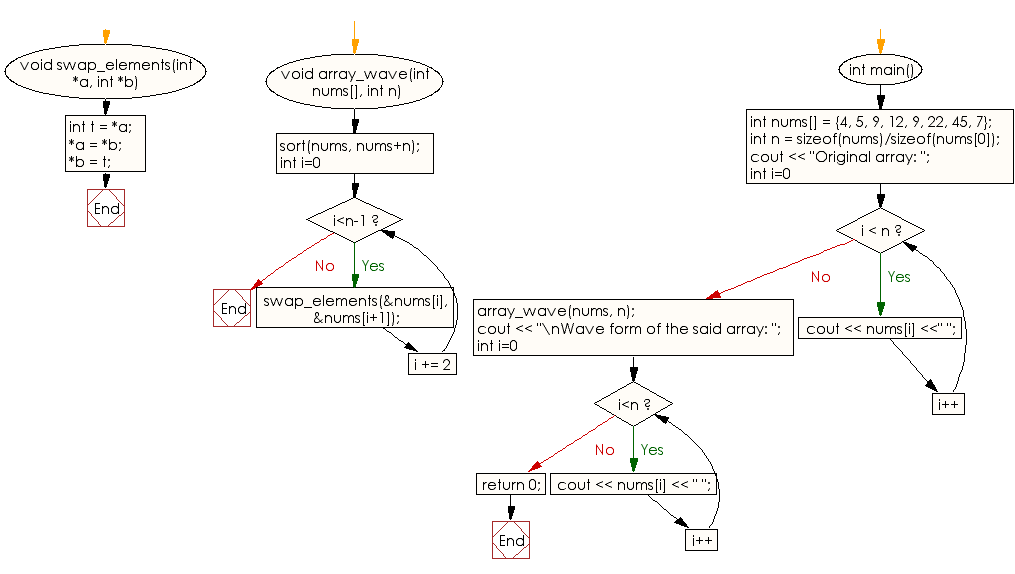﻿ C++ : Sort a given unsorted array of integers, in wave form# C++ Exercises: Sort a given unsorted array of integers, in wave form

## C++ Array: Exercise-9 with Solution

Write a C++ program to sort a given unsorted array of integers, in wave form.
Note: An array is in wave form when array >= array <= array >= array <= array >= . . . .

Sample Solution:

C++ Code :

``````#include<iostream>
#include<algorithm>
using namespace std;

void swap_elements(int *a, int *b)
{
int t = *a;
*a = *b;
*b = t;
}

void array_wave(int nums[], int n)
{
sort(nums, nums+n);

for (int i=0; i<n-1; i += 2)
swap_elements(&nums[i], &nums[i+1]);
}

int main()
{
int nums[] = {4, 5, 9, 12, 9, 22, 45, 7};
int n = sizeof(nums)/sizeof(nums);
cout << "Original array: ";
for (int i=0; i < n; i++)
cout << nums[i] <<" ";
array_wave(nums, n);
cout << "\nWave form of the said array: ";
for (int i=0; i<n; i++)
cout << nums[i] << " ";
return 0;
}
``````

Sample Output:

```Original array: 4 5 9 12 9 22 45 7
Wave form of the said array: 5 4 9 7 12 9 45 22
```

Flowchart:C++ Code Editor:

What is the difficulty level of this exercise?

Test your Programming skills with w3resource's quiz.

﻿